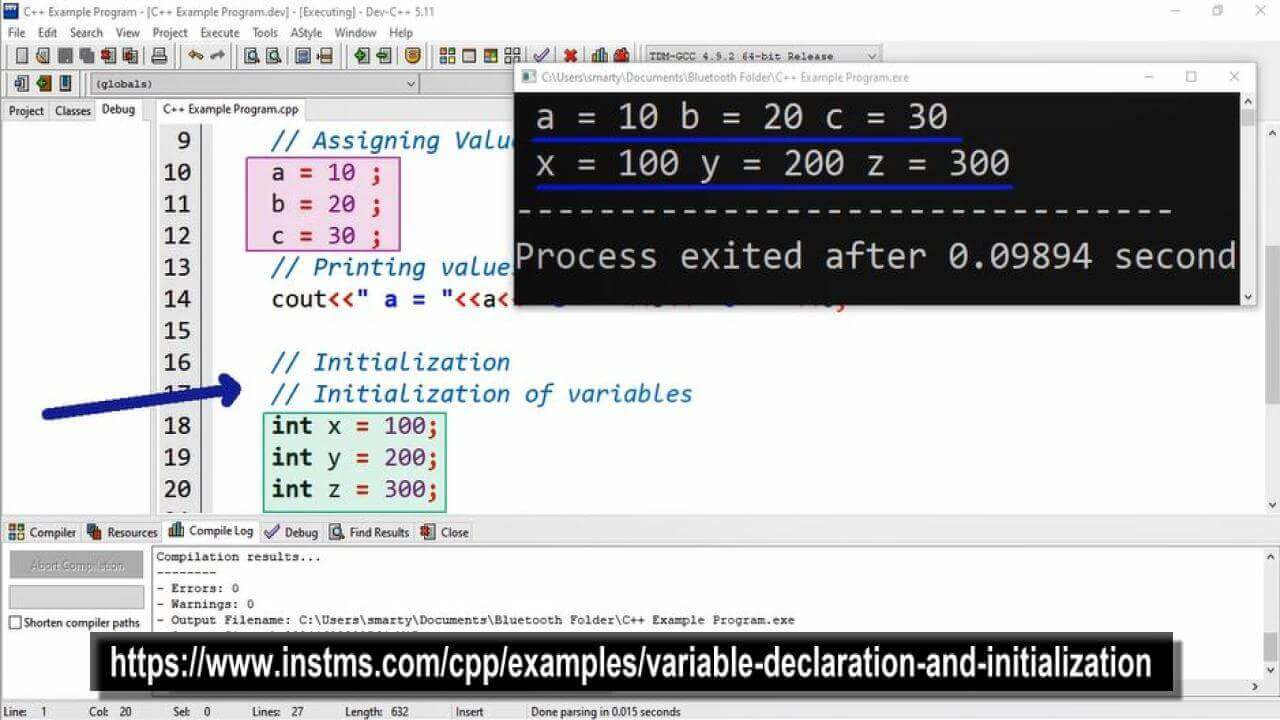# Variable Declaration And Initialization Program Example using CPP

## Source Code

#include <iostream>

using namespace std;

int main(int argc, char** argv) {

// Declaration
// Declaration of variables in single line
int a , b , c ;   // Variable can also be declare in multiple lines
// Assigning Values to variables
a = 10 ;
b = 20 ;
c = 30 ;
// Printing values of variables a,b,c
cout<<" a = "<<a<<" b = "<<b<<" c = "<<c;

// Initialization
// Initialization of variables
int x = 100;
int y = 200;
int z = 300;

// Printing values of variables x,y,z
cout<<"\n x = "<<x<<" y = "<<y<<" z = "<<z;

return 0;
}

##Working

In this program we declare three variables named a,b,c of int type in a single line. We can also declare variables individually using multiple declaration statements such as.

int a;

int b;

int c;

After declaration we assign values using assignment statement. Similarly initialization can also be done in single line, if we want to initialize all variables with same value such as.

int x = y = z = 10;

The above statement cannot be used if we want to initialize all variables with different values.